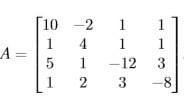# COSC 3416 assignment 5

Consider the matrix1. Use the algorithm on page 10 of lecture 16 to ﬁnd the LU factorization of A. Do your arithmetic exactly. Your answers will be fractions. Do not give your answers in decimal notation.

2. Use this LU factorization and the method described on page 17 of lecture 16 to ﬁnd A-1, the inverse of A. Do your arithmetic exactly. Your answers will be fractions. Do not give your answers in decimal notation.

3. Use the results of the theorem on page 11 of lecture 17 and the deﬁnition of the condition number of a matrix to ﬁnd the L1 condition number of A. Do your arithmetic exactly. Your answers will be fractions. Do not give your answers in decimal notation.

4. Use the results of the theorem on page 11 of lecture 17 and the deﬁnition of the condition number of a matrix to ﬁnd the L∞ condition number of A. Do your arithmetic exactly. Your answers will be fractions. Do not give your answers in decimal notation.